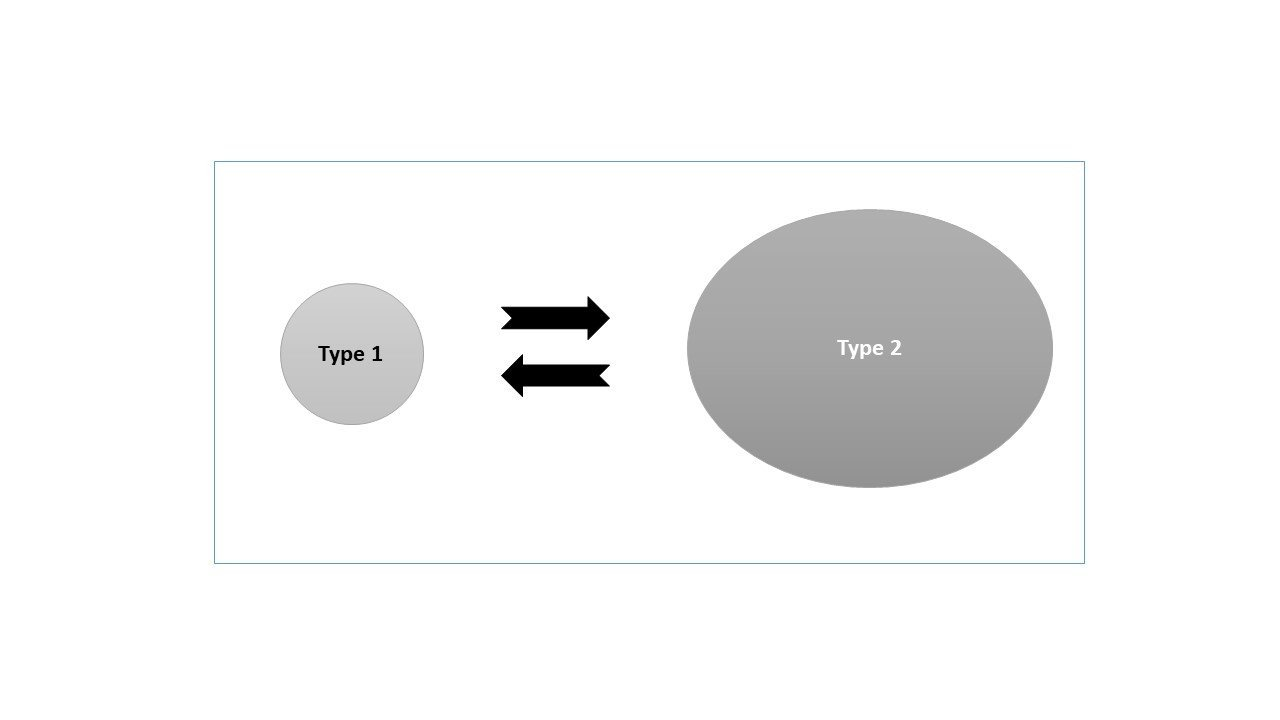Select Page

# Type Conversion in ABAP

by | Jun 3, 2018 | ABAP Programs

Home » SAP » ABAP » ABAP Programs » Type Conversion in ABAP

Preface – This post is part of the ABAP Programs series.

## Introduction

Type conversion in ABAB means converting the type of a variable. In ABAP programs sometime we need to convert a variable from one type to other. This can be achieved with the help of type conversion or type casting. The same concept is illustrated in the image given below:Type Conversion in ABAP Image Illustration

Note: If you are searching for type casting/conversion of ABAP Classes,  click here.

The given programs shows examples:

## 1. Direct assignment based conversion

```Data : lv_string type string value '123' ,
lv_num type num4.
lv_num = lv_string.
write : lv_num.
```

Explanation

The above code is explained line by line below:

1. In the first line, we have defined two variables of different data types: one is lv_string of type string with value “123” and another is lv_num of type num4.
2. This statement is used for type conversion. Here, we have directly assigned the value of lv_string to lv_num. Directly assigning the value causes conversion of the type.
3. Now, we will directly print the converted value as output. In actual programming, it is further used according to the requirement.

## 2. ABAP keyword based conversion

Note: Type conversion  can also be performed explicitly using the ABAP keyword for conversion operator CONV.

```DATA lv_text TYPE c LENGTH 255 VALUE 'ABC'.
DATA lv_xstr  TYPE xstring.
DATA(lv_xstr) = cl_abap_codepage=>convert_to( source = CONV #( lv_text ) ).
write : lv_xstr.```

Explanation

The above code is explained line by line below:

1. In the first and second line, we have defined two variables of different data types: one is lv_string of type string with value “ABC” and another is lv_xstr of type xstring.
2. This statement is used for type conversion. Here, we have used CONV keyword to perform conversion.
3. Now, we will directly print the converted value as output. In actual programming, it is further used according to the requirement.

## Author

•A Full Stack Developer with 10+ years of experience in technical content creation.

•